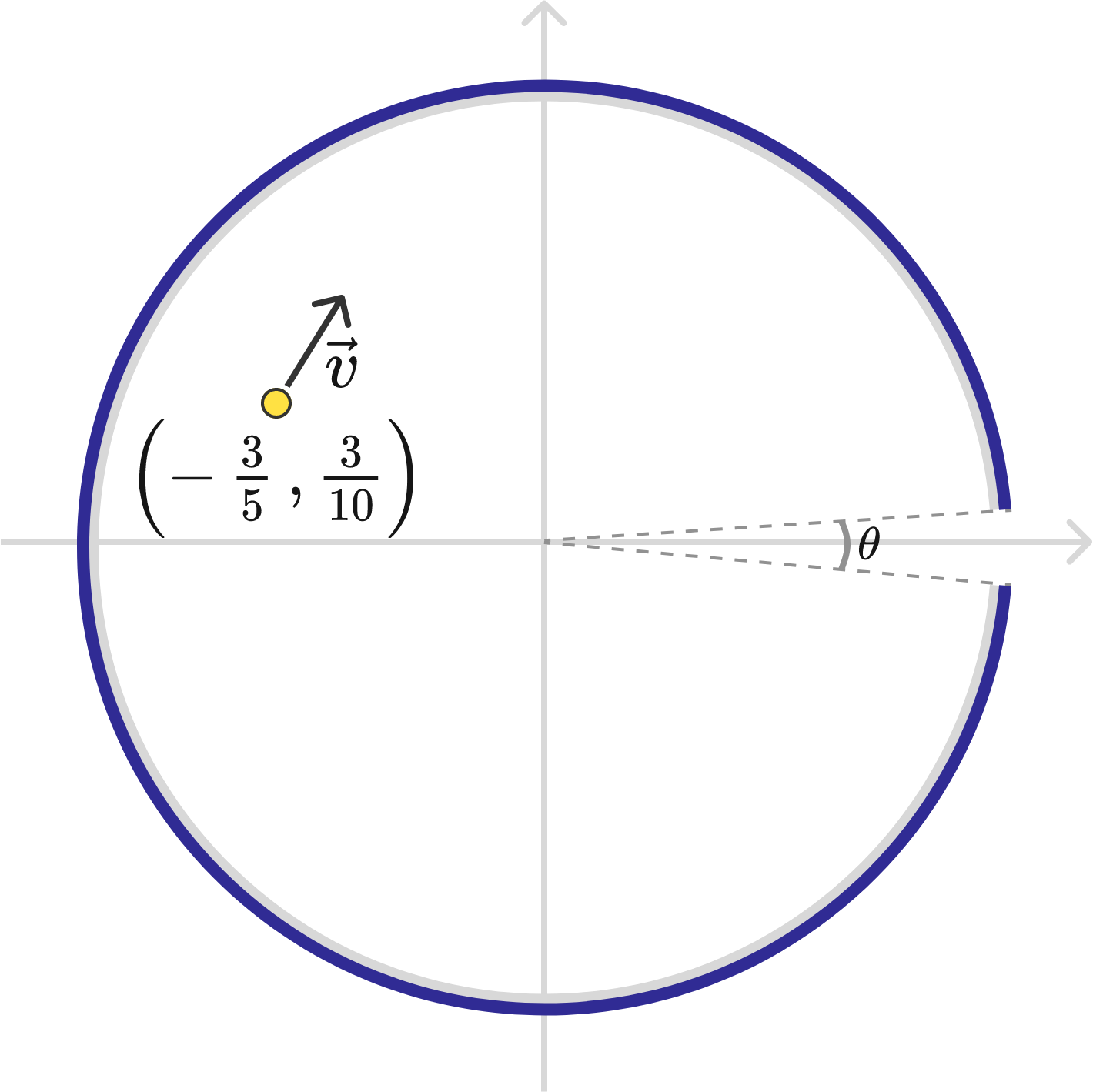# Bouncing Photon (Part 2)There is a photon traveling in the $xy$-plane. At $t = 0$, it is positioned at $(x,y) = \left(-\frac{3}{5} ,\frac{3}{10}\right)$ and it has a velocity $(v_x,v_y) = \left(\frac{1}{2},\frac{\sqrt{3}}{2}\right)$.

The photon is surrounded by a circular mirror of unit radius which is centered on the origin. There is no mirrored surface within the angle range $(-5^{\circ} \leq \theta \leq 5^{\circ})$, where $\theta$ is measured with respect to the $+x$ axis.

At what time does the photon exit the region enclosed by the circular mirror (to 1 decimal place)?

×